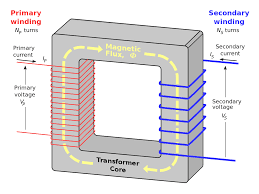-->

# Losses and Efficiency of Transformer - Electrical Diary

#### Due to the absence of movable parts, there is no mechanical loss(kinetic and other) occur in the transformer. there are only two types of loss that occur in transformer (a) Constant Loss (b) Variable Loss### Constant and Variable Loss In Transformer

As losses of any machine are defined as the difference of power out to power input. for a lossless machine, this power difference should be zero. But it is an ideal case, there is no device constructed in the world which converts all the input energy as useful work.

the transformer has only electrical loss i.e(Copper loss and Iron Loss)  it has no mechanical loss due to the absence of movable part.

### Copper Loss

when a load is connected across the secondary of the transformer then a load current starts to flow in the secondary and primary coil of the transformer. Due to this current I12R1 and I22R2 loss takes place in the primary and secondary coil of the transformer respectively.

This loss depends on the load current. if the load will increase then the loss will increase and if the load will decrease the loss will decrease. hence copper loss is called variable loss.

### Iron Loss

As we know the transfer of electrical energy from primary to secondary occurs as a function of the magnetic domain. without the presence of a magnetic field transfer of electrical energy from one circuit to another circuit can not take place.

Hence some part of input electrical energy is used by the core of the transformer to magnetize the core as magnetic energy. This magnetization of the core is irrespective of the load. Once the core is magnetized it will not vary either load will increase or decrease.

this part of input energy used by the core is taken under consideration as iron loss of the transformer. As this loss is constant irrespective of the load hence it is called constant loss also.
Iron losses are categories into two parts (a) Eddy Current Loss (b) Hysteresis loss

#### (a) Eddy Current Loss

Some part(very small) of Magnetic field produced by the primary and secondary of the transformer is linked with the iron core and induced an emf in the core of the transformer, this induced emf forced a current to flow in the core of the transformer.

this current is called eddy current, this current gives a loss in the core which is called eddy current loss. Eddy current loss is a function of the frequency of the magnetic field and magnetic field intensity.

(b) Hysteresis Loss

this loss is due to the reversal of magnetization in the transformer core. this loss depends on the dimension(volume), the grade of the iron, frequency of magnetic field, and value of magnetic flux density. this loss  can be given as
W= ηB1.6fV watt

where,
η = Steinmetz hysteresis constant
V = volume of the core in a cubic meter
this formula is called the Steinmetz formula

### Efficiency of Transformer

The efficiency of any machine is defined as the ratio of the output and input of the machine.it is unitless quantity tells about how much the given input energy is converted to the useful work.

The efficiency of any machine is denoted by the symbol (η).In the case of a transformer electrical energy is taken under consideration and the efficiency of a transformer is the ratio of primary side input electrical energy to the secondary side output energy.

Efficiency (η) = output/input

Input = output + loss
output = Input - loss

Efficiency (η) = (Input - loss)/input

Efficiency (η) = 1 -(loss/input)